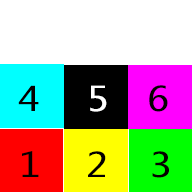# Change texture of cube sides

Hello everyone! Is there any way to change the texture of each side for a cube? Like minecaft? Please help me.

Thanks, any help is appriciated

Here’s the Unity 5 C# script equivalent of @robertbu’s script for mapping to the current cube primitive. I had to shuffle the UVs around until all of the sides looked right.

``````void Start () {

Mesh mesh = GetComponent<MeshFilter>().mesh;
Vector2[] UVs = new Vector2[mesh.vertices.Length];

// Front
UVs = new Vector2(0.0f, 0.0f);
UVs = new Vector2(0.333f, 0.0f);
UVs = new Vector2(0.0f, 0.333f);
UVs = new Vector2(0.333f, 0.333f);

// Top
UVs = new Vector2(0.334f, 0.333f);
UVs = new Vector2(0.666f, 0.333f);
UVs = new Vector2(0.334f, 0.0f);
UVs = new Vector2(0.666f, 0.0f);

// Back
UVs = new Vector2(1.0f, 0.0f);
UVs = new Vector2(0.667f, 0.0f);
UVs = new Vector2(1.0f, 0.333f);
UVs = new Vector2(0.667f, 0.333f);

// Bottom
UVs = new Vector2(0.0f, 0.334f);
UVs = new Vector2(0.0f, 0.666f);
UVs = new Vector2(0.333f, 0.666f);
UVs = new Vector2(0.333f, 0.334f);

// Left
UVs = new Vector2(0.334f, 0.334f);
UVs = new Vector2(0.334f, 0.666f);
UVs = new Vector2(0.666f, 0.666f);
UVs = new Vector2(0.666f, 0.334f);

// Right
UVs = new Vector2(0.667f, 0.334f);
UVs = new Vector2(0.667f, 0.666f);
UVs = new Vector2(1.0f, 0.666f);
UVs = new Vector2(1.0f, 0.334f);

mesh.uv = UVs;
}
``````

I also made a big, clean version of @robertbu’s atlas, using words instead of numbers.
Here is my version of the atlas:

As @Eric5h5 mentions, typically the UV mapping is done in a 3D graphics app. And that is how its been done for resources I’ve used. But in theory the UV map of a built in-cube can be mapped to an atlas. I wanted to see how hard it would be.

Here is the sample atlas I used:And here is the sample script. Attach the script to a cube. Create a material using the above texture and also add it to a cube.

``````#pragma strict

function Start () {
var mf = GetComponent(MeshFilter);
var mesh : Mesh;
if (mf != null)
mesh = mf.mesh;

if (mesh == null || mesh.uv.Length != 24) {
Debug.Log("Script needs to be attached to built-in cube");
return;
}

var uvs = mesh.uv;

// Front
uvs  = Vector2(0.0, 0.0);
uvs  = Vector2(0.333, 0.0);
uvs  = Vector2(0.0, 0.333);
uvs  = Vector2(0.333, 0.333);

// Top
uvs  = Vector2(0.334, 0.0);
uvs  = Vector2(0.666, 0.0);
uvs  = Vector2(0.334, 0.333);
uvs  = Vector2(0.666, 0.333);

// Back
uvs = Vector2(0.667, 0.0);
uvs = Vector2(1.0, 0.0);
uvs  = Vector2(0.667, 0.333);
uvs  = Vector2(1.0, 0.333);

// Bottom
uvs = Vector2(0.0, 0.334);
uvs = Vector2(0.333, 0.334);
uvs = Vector2(0.0, 0.666);
uvs = Vector2(0.333, 0.666);

// Left
uvs = Vector2(0.334, 0.334);
uvs = Vector2(0.666, 0.334);
uvs = Vector2(0.334, 0.666);
uvs = Vector2(0.666, 0.666);

// Right
uvs = Vector2(0.667, 0.334);
uvs = Vector2(1.00, 0.334);
uvs = Vector2(0.667, 0.666);
uvs = Vector2(1.0, 0.666);

mesh.uv = uvs;
}
``````

You need to make a texture atlas, then create a cube in a 3D app and UV map the sides as appropriate for your texture atlas. Alternately, create each side as a submesh, and use different materials with different textures for each submesh. This is slower (more draw calls) but won’t have any mipmap bleeding issues.